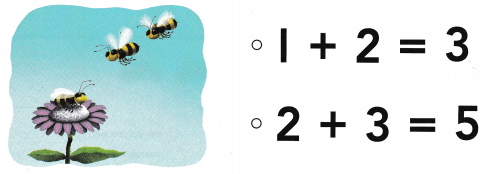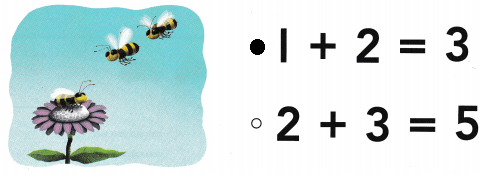# Texas Go Math Kindergarten Module 11 Assessment Answer Key

Refer to our Texas Go Math Kindergarten Answer Key Pdf to score good marks in the exams. Test yourself by practicing the problems from Texas Go Math Kindergarten Module 11 Assessment Answer Key.

## Texas Go Math Kindergarten Module 11 Assessment Answer Key

Concepts and Skills

DIRECTIONS: 1. Two cows are in a meadow. One more cow comes into the meadow. Write the numbers to show the cows being added. (TEKS K.3.A) 2-3. Listen to the addition word problem. Circle the set you start with. How many are being added to the set? How many are there now? Trace and write the numbers and symbols to complete the addition sentence. (TEKS K.3.B)

Question 1.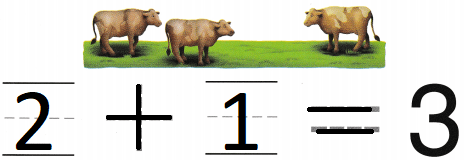Explanation:
Two cows are in a meadow.
One more cow comes into the meadow.
2 + 1 = 3
so, 3 cows are in the meadow.

Question 2.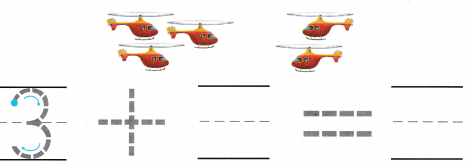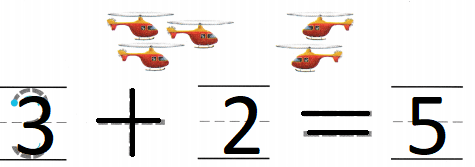Explanation:
There are 3 aero planes flying in the sky
2 more aero planes joined them
3 + 2 = 5
so, 5 aero planes flying in the sky.

Question 3.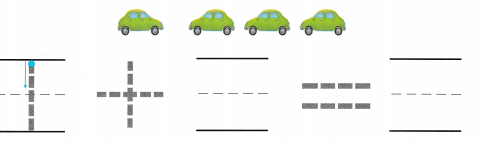Explanation:
1 car started racing
then 3 more cars joined them
1 + 3 = 4
so, 4 cars are in racing

DIRECTIONS: 4. There are four ducks swimming in a pond. One more duck comes. How many ducks are there? Write the number sentence. (TEKS K.3.B) 5. There are two squirrels by a tree. There are two more squirrels. How many squirrels are there altogether? Write the number sentence. 6. Choose the correct answer. One bee is on a flower. Two more bees come. Act out the problem. What does the picture show? (TEKS K.3.C)

Question 4.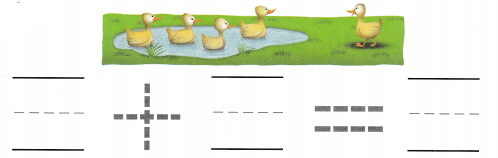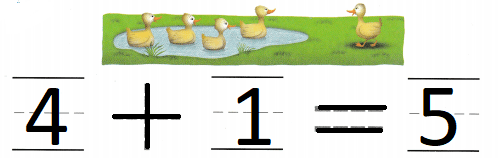Explanation:
There are four ducks swimming in a pond.
One more duck comes.
4 + 1 = 5
so, 5 ducks are in the pond.

Question 5.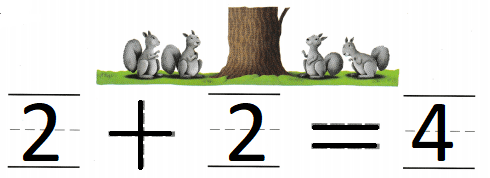Explanation:
There are two squirrels by a tree.
There are two more squirrels.
2 + 2 = 4
so, 4 squirrels are near the tree.

Question 6.
Texas Test Prep